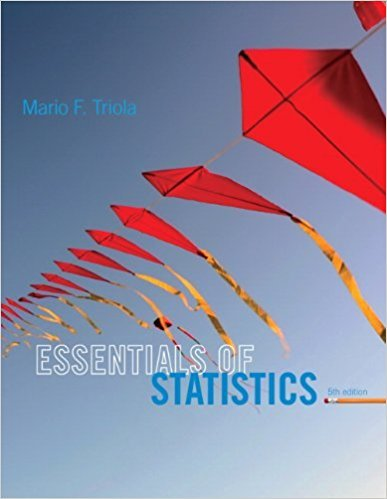×
×

# Solutions for Chapter 2.2: Frequency Distributions## Full solutions for Essentials of Statistics | 5th Edition

ISBN: 9780321924599Solutions for Chapter 2.2: Frequency Distributions

Solutions for Chapter 2.2
4 5 0 392 Reviews
11
2
##### ISBN: 9780321924599

Since 34 problems in chapter 2.2: Frequency Distributions have been answered, more than 14427 students have viewed full step-by-step solutions from this chapter. This textbook survival guide was created for the textbook: Essentials of Statistics, edition: 5. Essentials of Statistics was written by and is associated to the ISBN: 9780321924599. Chapter 2.2: Frequency Distributions includes 34 full step-by-step solutions. This expansive textbook survival guide covers the following chapters and their solutions.

Key Statistics Terms and definitions covered in this textbook
• Binomial random variable

A discrete random variable that equals the number of successes in a ixed number of Bernoulli trials.

• Coeficient of determination

See R 2 .

• Conditional probability

The probability of an event given that the random experiment produces an outcome in another event.

• Conditional probability density function

The probability density function of the conditional probability distribution of a continuous random variable.

• Correlation

In the most general usage, a measure of the interdependence among data. The concept may include more than two variables. The term is most commonly used in a narrow sense to express the relationship between quantitative variables or ranks.

• Covariance

A measure of association between two random variables obtained as the expected value of the product of the two random variables around their means; that is, Cov(X Y, ) [( )( )] =? ? E X Y ? ? X Y .

• Deining relation

A subset of effects in a fractional factorial design that deine the aliases in the design.

• Deming

W. Edwards Deming (1900–1993) was a leader in the use of statistical quality control.

• Deming’s 14 points.

A management philosophy promoted by W. Edwards Deming that emphasizes the importance of change and quality

• Designed experiment

An experiment in which the tests are planned in advance and the plans usually incorporate statistical models. See Experiment

• Discrete uniform random variable

A discrete random variable with a inite range and constant probability mass function.

• Erlang random variable

A continuous random variable that is the sum of a ixed number of independent, exponential random variables.

• Error mean square

The error sum of squares divided by its number of degrees of freedom.

• Event

A subset of a sample space.

• F-test

Any test of signiicance involving the F distribution. The most common F-tests are (1) testing hypotheses about the variances or standard deviations of two independent normal distributions, (2) testing hypotheses about treatment means or variance components in the analysis of variance, and (3) testing signiicance of regression or tests on subsets of parameters in a regression model.

• False alarm

A signal from a control chart when no assignable causes are present

• Fraction defective

In statistical quality control, that portion of a number of units or the output of a process that is defective.

• Gamma random variable

A random variable that generalizes an Erlang random variable to noninteger values of the parameter r

• Generator

Effects in a fractional factorial experiment that are used to construct the experimental tests used in the experiment. The generators also deine the aliases.

• Geometric random variable

A discrete random variable that is the number of Bernoulli trials until a success occurs.

×